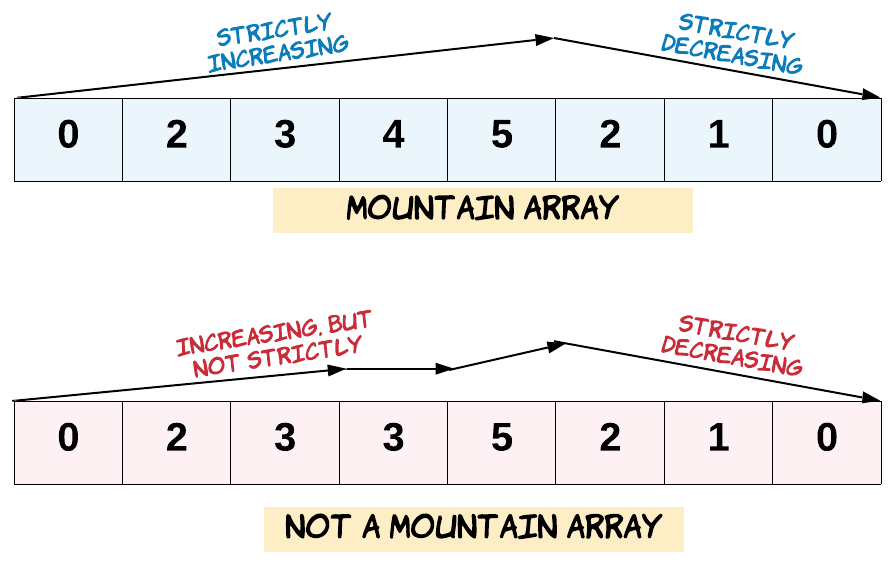# Question

Formatted question description: https://leetcode.ca/all/941.html

Given an array A of integers, return true if and only if it is a *valid mountain array*.
Recall that A is a mountain array if and only if:

A.length >= 3
There exists some i with 0 < i < A.length - 1 such that:
A < A < ... A[i-1] < A[i]
A[i] > A[i+1] > ... > A[A.length - 1]Example 1:

Input: [2,1]
Output: false
Example 2:

Input: [3,5,5]
Output: false
Example 3:

Input: [0,3,2,1]
Output: true
Note:

0 <= A.length <= 10000
0 <= A[i] <= 10000



# Algorithm

This question defines a mountain-shaped array, the length is greater than or equal to 3, and there is a peak value, the numbers on the left and right sides must be strictly decreasing, and no equal values are allowed.

That is to say, traversing from the beginning must be strictly increasing until reaching the peak, and then strictly decreasing to the end, then the while loop can be carried out from the beginning, if the current number is less than the number on the right, then i will increment by 1.

In order to avoid overflow, i only can traverse to the penultimate number, so that when the loop ends, the number pointed to by i is greater than or equal to the number on the right, which is a potential peak value. Of course, it cannot be equal here, but there is no need to judge at this time.

The same operation is reversed, j traverses from the last number to the second number. If the current number is less than the number on the left, j is decremented by 1, so that after the loop ends, the number pointed to by j is greater than or equal to the number on the left It is also a potential peak. The next step is to compare whether the two peaks point to the same number. At the same time, the number pointed to by i cannot be the first number, and the number pointed to by j cannot be the last number, because there must be uphill and downhill, see code as follows:

# Code

C++

class Solution {
public:
bool validMountainArray(vector<int>& A) {
int n = A.size(), i = 0, j = n - 1;
while (i < n - 1 && A[i] < A[i + 1]) ++i;
while (j > 0 && A[j - 1] > A[j]) --j;
return i > 0 && j < n - 1 && i == j;
}
};


• class Solution {
public boolean validMountainArray(int[] A) {
if (A == null || A.length < 3)
return false;
int length = A.length;
if (A >= A || A[length - 2] <= A[length - 1])
return false;
int topIndex = -1;
for (int i = 1; i < length; i++) {
int difference = A[i] - A[i - 1];
if (difference == 0)
return false;
else if (difference < 0) {
topIndex = i - 1;
break;
}
}
for (int i = topIndex + 1; i < length; i++) {
int difference = A[i] - A[i - 1];
if (difference >= 0)
return false;
}
return true;
}
}

############

class Solution {
public boolean validMountainArray(int[] arr) {
int n = arr.length;
if (n < 3) {
return false;
}
int l = 0, r = n - 1;
while (l + 1 < n - 1 && arr[l] < arr[l + 1]) {
++l;
}
while (r - 1 > 0 && arr[r] < arr[r - 1]) {
--r;
}
return l == r;
}
}

• // OJ: https://leetcode.com/problems/valid-mountain-array/
// Time: O(N)
// Space: O(1)
class Solution {
public:
bool validMountainArray(vector<int>& A) {
int i = 1, N = A.size();
while (i < N && A[i] > A[i - 1]) ++i;
if (i == 1 || i == N) return false;
while (i < N && A[i] < A[i - 1]) ++i;
return i == N;
}
};

• class Solution:
def validMountainArray(self, arr: List[int]) -> bool:
n = len(arr)
if n < 3:
return False
l, r = 0, n - 1
while l + 1 < n - 1 and arr[l] < arr[l + 1]:
l += 1
while r - 1 > 0 and arr[r] < arr[r - 1]:
r -= 1
return l == r

############

class Solution:
def validMountainArray(self, A):
"""
:type A: List[int]
:rtype: bool
"""
N = len(A)
if N < 3:
return False
i = 0
while i < N - 1:
if A[i] < A[i + 1]:
i += 1
else:
break
if i == 0 or i == N - 1: return False
while i < N - 1:
if A[i] > A[i + 1]:
i += 1
else:
break
return i == N - 1

• func validMountainArray(arr []int) bool {
n := len(arr)
if n < 3 {
return false
}
l, r := 0, n-1
for l+1 < n-1 && arr[l] < arr[l+1] {
l++
}
for r-1 > 0 && arr[r] < arr[r-1] {
r--
}
return l == r
}